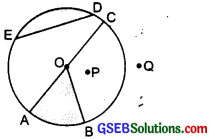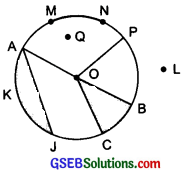# GSEB Solutions Class 6 Maths Chapter 4 Basic Geometrical Ideas Ex 4.6

Gujarat Board GSEB Textbook Solutions Class 6 Maths Chapter 4 Basic Geometrical Ideas Ex 4.6 Textbook Questions and Answers.

## Gujarat Board Textbook Solutions Class 6 Maths Chapter 4 Basic Geometrical Ideas Ex 4.6

Question 1.
From the figure, identify:(a) the centre of circle
(c) a diameter
(d) a chord
(e) two points in the interior
(f) a point in the exterior
(g) a sector
(h) a segment
Solution:
In the given figure, we have:
(a) The centre of the circle is O.
(b) The three radii of the circle are:
(i) $$\overline{\mathrm{OA}}$$
(ii) $$\overline{\mathrm{OB}}$$
(iii) $$\overline{\mathrm{OC}}$$
(c) A diameter of the circle is $$\overline{\mathrm{AC}}$$
(d) A chord of the circle is $$\overline{\mathrm{ED}}$$.
(e) Two points in the interior of the circle are O and P.
(f) One point in the exterior of the circle is Q.
(g) A sector of the circle is shaded as OBA.
(h) A segment of the circle is shaded as ED.Question 2.
(a) Is every diameter of a circle also a chord?
(b) Is every chord of a circle also a diameter?
Solution:
(a) Yes, the diameter is the longest chord of a circle.
(b) No, every chord of a circle is not also a diameter.Question 3.
Draw any circle and mark
(a) it’s centre
(c) a diameter
(d) a sector
(e) a segment
(f) a point in its interior
(g) a point in its exterior
(h) an are
Solution:
(a) Centre of the circle is O.
(b) $$\overline{\mathrm{OP}}$$ is a radius of the circle.
(c) $$\overline{\mathrm{AB}}$$ is a diameter of the circle.
(d) OBC is a sector (shaded part).
(e) AJK (shaded part) is a segment of the circle.
(f) The point Q is in the interior of the circle.
(g) The point L is in the exterior of the circle.
(h) $$\overline{\mathrm{MN}}$$ is an are of the circle.Question 4.
Say true or false:
(a) Two diameters of a circle will necessarily intersect.
(b) The centre of a circle is always in its interior
Solution:
(a) Yes, it is a true statement.
(b) Yes, it is a true statement.